# 6 Results

View
Selected filters:
• Functions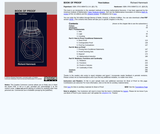Rating

This book will initiate you into an esoteric world. You will learn and apply the methods of thought that mathematicians use to verify theorems, explore mathematical truth and create new mathematical theories. This will prepare you for advanced mathematics courses, for you will be better able to understand proofs, write your own proofs and think critically and inquisitively about mathematics.

Subject:
Functions
Material Type:
Textbook
Provider:
Virginia Commonwealth University
Author:
Richard Hammack
01/01/2009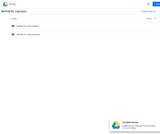Conditions of Use:
No Strings Attached
Rating

This course is an introduction to contemporary calculus and is the first of a three-part sequence. In this course students explore the concepts, applications, and techniques of Calculus - the mathematics of change. Calculus has wide-spread application in science, economics and engineering, and is a foundation college course for further work in these areas. This is a required class for most science and mathematics majors.Login: guest_oclPassword: ocl

Subject:
Calculus
Functions
Material Type:
Full Course
Homework/Assignment
Lecture Notes
Lesson Plan
Syllabus
Provider:
Washington State Board for Community & Technical Colleges
Provider Set:
Open Course Library
10/31/2011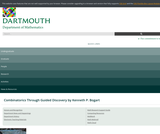Conditions of Use:
Rating

Subject:
Mathematics
Functions
Material Type:
Textbook
Provider:
Dartmouth College
Author:
Kenneth Bogart
01/01/2004Conditions of Use:
Remix and Share
Rating

This is a text for a two-term course in introductory real analysis for junior or senior mathematics majors and science students with a serious interest in mathematics. Prospective educators or mathematically gifted high school students can also benefit from the mathematical maturity that can be gained from an introductory real analysis course.

The book is designed to fill the gaps left in the development of calculus as it is usually presented in an elementary course, and to provide the background required for insight into more advanced courses in pure and applied mathematics. The standard elementary calculus sequence is the only specific prerequisite for Chapters 1–5, which deal with real-valued functions. (However, other analysis oriented courses, such as elementary differential equation, also provide useful preparatory experience.) Chapters 6 and 7 require a working knowledge of determinants, matrices and linear transformations, typically available from a first course in linear algebra. Chapter 8 is accessible after completion of Chapters 1–5.

Subject:
Mathematics
Calculus
Functions
Material Type:
Textbook
Provider:
Trinity University
Provider Set:
Digital Commons @Trinity University
Author:
William Trench
12/01/2013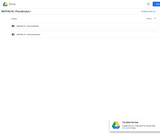Conditions of Use:
No Strings Attached
Rating

This course will cover families of functions, their properties, graphs and applications. These functions include: polynomial, rational, exponential, logarithmic functions and combinations of these. We will solve related equations and inequalities and conduct data analysis, introductory mathematical modeling and develop competency with a graphing calculator.Login: guest_oclPassword: ocl

Subject:
Functions
Material Type:
Full Course
Homework/Assignment
Lecture Notes
Lesson Plan
Syllabus
Provider:
Washington State Board for Community & Technical Colleges
Provider Set:
Open Course Library
10/31/2011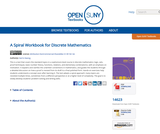Conditions of Use:
Remix and Share
Rating

This is a text that covers the standard topics in a sophomore-level course in discrete mathematics: logic, sets, proof techniques, basic number theory, functions, relations, and elementary combinatorics, with an emphasis on motivation. It explains and clarifies the unwritten conventions in mathematics, and guides the students through a detailed discussion on how a proof is revised from its draft to a final polished form. Hands-on exercises help students understand a concept soon after learning it. The text adopts a spiral approach: many topics are revisited multiple times, sometimes from a different perspective or at a higher level of complexity. The goal is to slowly develop students’ problem-solving and writing skills.

Subject:
Mathematics
Functions
Numbers and Operations
Material Type:
Textbook
Provider:
State University of New York
Provider Set:
OpenSUNY Textbooks
Author:
Harris Kwong, SUNY Fredonia